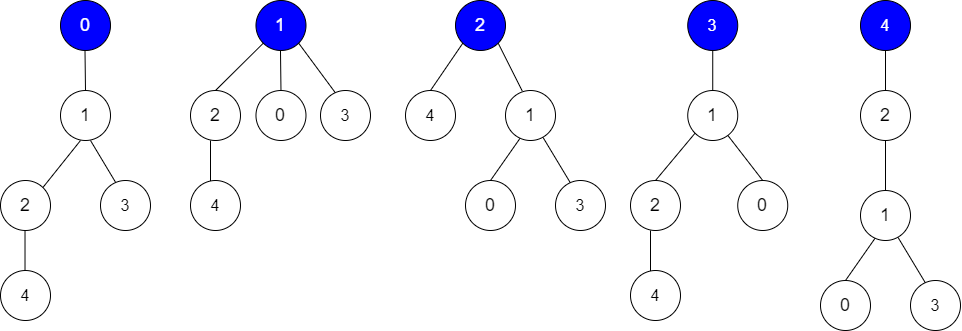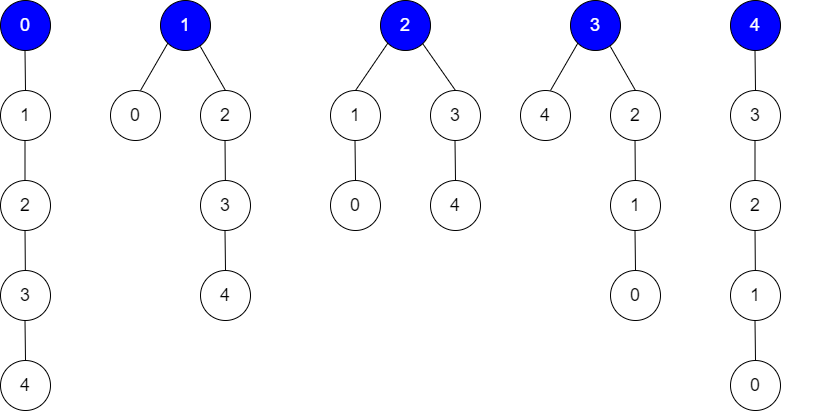2581. Count Number of Possible Root Nodes
Hard
187
3

Alice has an undirected tree with `n` nodes labeled from `0` to `n - 1`. The tree is represented as a 2D integer array `edges` of length `n - 1` where `edges[i] = [ai, bi]` indicates that there is an edge between nodes `ai` and `bi` in the tree.

Alice wants Bob to find the root of the tree. She allows Bob to make several guesses about her tree. In one guess, he does the following:

• Chooses two distinct integers `u` and `v` such that there exists an edge `[u, v]` in the tree.
• He tells Alice that `u` is the parent of `v` in the tree.

Bob's guesses are represented by a 2D integer array `guesses` where `guesses[j] = [uj, vj]` indicates Bob guessed `uj` to be the parent of `vj`.

Alice being lazy, does not reply to each of Bob's guesses, but just says that at least `k` of his guesses are `true`.

Given the 2D integer arrays `edges`, `guesses` and the integer `k`, return the number of possible nodes that can be the root of Alice's tree. If there is no such tree, return `0`.

Example 1:```Input: edges = [[0,1],[1,2],[1,3],[4,2]], guesses = [[1,3],[0,1],[1,0],[2,4]], k = 3
Output: 3
Explanation:
Root = 0, correct guesses = [1,3], [0,1], [2,4]
Root = 1, correct guesses = [1,3], [1,0], [2,4]
Root = 2, correct guesses = [1,3], [1,0], [2,4]
Root = 3, correct guesses = [1,0], [2,4]
Root = 4, correct guesses = [1,3], [1,0]
Considering 0, 1, or 2 as root node leads to 3 correct guesses.

```

Example 2:```Input: edges = [[0,1],[1,2],[2,3],[3,4]], guesses = [[1,0],[3,4],[2,1],[3,2]], k = 1
Output: 5
Explanation:
Root = 0, correct guesses = [3,4]
Root = 1, correct guesses = [1,0], [3,4]
Root = 2, correct guesses = [1,0], [2,1], [3,4]
Root = 3, correct guesses = [1,0], [2,1], [3,2], [3,4]
Root = 4, correct guesses = [1,0], [2,1], [3,2]
Considering any node as root will give at least 1 correct guess.

```

Constraints:

• `edges.length == n - 1`
• `2 <= n <= 105`
• `1 <= guesses.length <= 105`
• `0 <= ai, bi, uj, vj <= n - 1`
• `ai != bi`
• `uj != vj`
• `edges` represents a valid tree.
• `guesses[j]` is an edge of the tree.
• `guesses` is unique.
• `0 <= k <= guesses.length`
Accepted
3.3K
Submissions
6.8K
Acceptance Rate
48.2%

Seen this question in a real interview before?
1/4
Yes
No

Discussion (0)

Related Topics